Trial ends in

A subscription to JoVE is required to view this content.
You will only be able to see the first 20 seconds.

Angular Momentum
Science Education (Physics I)
Force and Acceleration
Science Education (Physics I)
Equilibrium and Free-body Diagrams

Angular Momentum

Overview

Source: Nicholas Timmons, Asantha Cooray, PhD, Department of Physics & Astronomy, School of Physical Sciences, University of California, Irvine, CA

Angular momentum is defined as the product of the moment of inertia and the angular velocity of the object. Like its linear analog, angular momentum is conserved, meaning that the total angular momentum of a system will not change if there are no external torques on the system. A torque is the rotational equivalent of a force. Because it is a conserved, angular momentum is an important quantity in physics.

The goal of this experiment is to measure the angular momentum of a rotating rod and to use the conservation of angular momentum to explain two rotational demonstrations.

Principles

Angular momentum can be written as: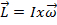, (Equation 1)

whereis the moment of inertia and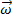is the angular velocity. The moment of inertia is the rotational analog of mass for linear motion. It is related to the mass distribution of a rotating object and the axis of rotation. The larger the moment of inertia, the more torque is needed to cause an angular acceleration on an object. The right-hand rule can be used to determine the direction of angular momentum. When the fingers of the right hand curl in the direction of rotation, the extended thumb points in the direction of angular momentum.

A torque is defined as the product of a force applied at some distance from an axis of rotation: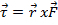, (Equation 2)

where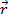is the distance to the axis of rotation and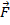is the force applied. If a torque acts on an object, the angular velocity of that object will change, along with its angular momentum. If the sum of the torques on an object is equal to zero, then the total angular momentum will be conserved and will have the same final value as it did initially.

A fun example of the conservation of angular momentum can be demonstrated with a bike wheel and a rotating chair. The wheel and the person in the chair constitute a system with some angular momentum. If the person applies a torque to spin the wheel, with the axis pointing vertically, the system will have gained angular momentum. If the person then flips the spinning wheel over, she will begin to spin in her chair in the opposite direction of the spinning wheel. Here, the system had angular momentum, with its direction determined by the right-hand rule. When the wheel was flipped, the angular momentum of the system changed direction. Because of conservation, the chair began to rotate in the opposite direction so that the total angular momentum of the system was equal to that of the system before the wheel was flipped.

Another demonstration of the conservation of angular momentum can be done with a spinning chair and two weights. If the weights are held out at arm's length while the chair is spinning and then are brought in close to the chest, there will be an increase in angular velocity. This happens because bringing the weights nearer to the axis of rotation decreases the moment of inertia of the system. If there is no more force acting to spin the chair, then the torque on the system is zero. The angular momentum must remain constant, as there are no torques, and the only way for that to happen is for the angular speed to increase.

In this experiment, a rotating rod is connected to a falling weight. The falling weight will provide a torque on the rod, and the angular momentum will be measured at two points: first when the weight has fallen halfway and then again once the weight reaches the end of the string. See Figure 1 for an image of the experimental setup.

The moment of inertia of a spinning rod is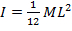, where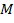is the mass of the rod and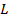is the length. These quantities can be measured before the experiment takes place. To find the angular velocity, the rotational kinematic equations will be used: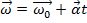. (Equation 3)

Equation 3 states that the final angular velocityis equal to the initial angular velocityplus the angular acceleration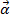, multiplied by time. Because the rod will begin at rest,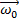will be equal to zero. The angular accelerationis defined by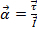, where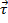is the torque andis the moment of inertia. The torque is the cross product of the distance from the axis of rotation to the force, and the force of the weight causing a tension in the string, which causes the rod to rotate:. That force acting on the pulley is equal to the force on the weight: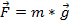, where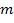is the mass and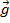is the acceleration due to gravity. The radiusof the torque will be the distance from the wound string to the axis of rotation.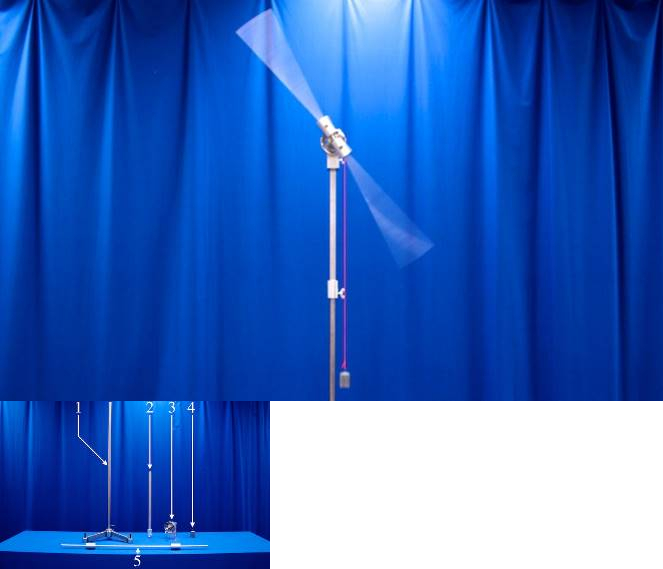Figure 1. Experimental setup. Inset: 1) large ring stand, 2) extender, 3) rotating assembly, 4) weight, and 5) torque bar.

Procedure

1. Test the theory of the conservation of angular momentum with the bike wheel.

1. While sitting in a chair that can freely rotate, start spinning the bike wheel and then hold it by the handles so that its direction of angular momentum is vertical.
2. While holding the wheel by the two handles, flip the wheel over so that its angular momentum points in the opposite direction. Notice how the chair will begin to rotate.

2. Test the theory of the conservation of angular momentum with two weights.

1. While sitting in a chair that can freely rotate, hold two weights out at arm's length.
2. Have a partner get the chair spinning and then bring the weights in close to the chest. Notice the increased speed of rotation of the chair.

3. Measure the change of angular momentum in the spinning rod.

1. Measure the length of the rod and its mass. Using a meter stick, measure the halfway point of the falling weight and mark the vertical beam with tape in order to have a reference. Calculate the moment of inertia of the rod.
2. Add 200 g to the end of the string and wind it up to the top. Take notice of where the halfway point of the string is located.
3. Release the weight and measure the amount of time it takes to get to the halfway mark and then again to the bottom. Do this three times and take the average values. Calculate the angular momentum at both points.
4. Increase the weight at the end of the string to 500 g and repeat step 3.3.
5. Increase the weight to 1,000 g and repeat step 3.3.

A spinning mass has the property of angular momentum and conservation of angular momentum is central to solving problems in rotational dynamics.

As explained in another video of this collection, an object's linear momentum does not change, that is Δp is zero until a net external force is applied.

The same conservation principle applies to angular momentum, denoted by the letter L. So ΔL is also zero until a net external torque is applied.

Here, we will first explain the concept of angular momentum and show how it is conserved using different examples. Then the video will demonstrate a lab experiment involving measurement of angular momentum for a spinning rod.

To understand angular momentum, let's consider a ball attached to string undergoing a rotational motion about an axis. The magnitude of angular momentum of this ball 'L' is r - the radius of the circle - times p, which is the translational momentum. Now p is mass times velocity, where velocity is the tangential velocity. The tangential velocity is the angular velocity 'ω' times r. The direction of angular momentum is given by the right-hand rule. If you curl the fingers of the right hand in the direction of rotation, then the extended thumb points in the direction of the angular momentum of the system.

Based on this formula and the principle of angular momentum conservation, we can predict that in the absence of net external torque, if r is reduced ω would increase, and if r is increased ω would decrease.

This principle of angular momentum conservation is evident in figure skating. With the arms out the skater rotates at one speed, but as soon as they bring their arms in, the rotation speed increases significantly.

Now that we have reviewed the principle of angular momentum conservation, let's see it in action in a physics lab. For the first demonstration, sit in a chair that can rotate freely and hold two weights out at arm's length. Ask another person to spin the chair. While spinning, bring the weights close to the chest and notice how the chair's speed of rotation increases.

As with the spinning ice skater, when the weights are held far from the body, the person on the chair has a high moment of inertia due to a relatively bigger r. Bringing the weights close to the body reduces the system's moment of inertia, and thus due to conservation of angular momentum, the speed of rotation increases.

For the second demonstration, again sit in a chair that can rotate freely and hold a bicycle wheel by the handles so its axis is vertical. Then spin the wheel counterclockwise, keeping the chair stationary. By the right-hand rule, the direction of the wheel's angular momentum vector is vertical, pointing up.

Flip the wheel so it is spinning clockwise when the axis is vertical again. Now its angular momentum points down. Notice how the chair spins in response.

The bicycle wheel, the person holding it and the chair make up a system of multiple objects. When the wheel alone is spinning, this system has a certain total angular momentum. Although the person holding the wheel applies a torque to flip it over, this torque originates within the system and the net external torque is zero.

With no external applied torque, angular momentum is conserved, meaning it does not change. Flipping the wheel reverses the direction of its angular momentum. In order to keep the total amount of angular momentum in the system conserved, the person and chair must spin, so that their combined angular momentum vector opposes that of the wheel.

As a result, the total angular momentum of the person, chair and flipped wheel must have the same magnitude and be in the same direction as the angular momentum of the wheel in its original position.

Next, let's see an experiment involving measurement of angular momentum of a spinning rod. For this, a falling weight pulls a string wound around an axle. The magnitude of the resulting torque is the tension in the string times the radius of the axle. This torque spins the axle, causing rotational acceleration of the rod attached to it. The rod's moment of inertia can be calculated from its mass M and length L.

The spinning rod's angular acceleration is equal to this torque divided by the rod's moment of inertia. With this information, it is possible to calculate angular velocity at any time from the equations for rotational kinematics.

Finally, using the rod's moment of inertia and angular velocity, the spinning rod's angular momentum will be determined at two points: when the weight has fallen halfway and when it has reached the end of its travel.

Before starting the experiment, measure the rod's length and mass then calculate its moment of inertia. Use a meter stick to determine the halfway point of the weight's downward travel. Mark this point with tape on the vertical beam. Attach 200 grams to the end of the string and wind it until the weight reaches the top.

Release the weight and measure the amount of time to reach the halfway point and the amount of time to reach the bottom. Record the results. Do this three times and use the average values to calculate the angular momentum at both points.

Increase the weight on the string to 500 grams. Perform the procedure four times and record the results. Then increase the weight to 1000 grams, repeat the procedure and record the results.

As the mass of the falling weight increases, the torque and angular acceleration on the axle of the spinning rod should increase proportionally. Theoretically, at any given time both the angular velocity and angular momentum should increase proportionally with this torque.

At any given distance that the weight had fallen, the angular momentum of the spinning rod should have been proportional to the square root of the weight's mass. The experiment showed that the angular momenta with the 500 gram weight were indeed approximately 1.6-or the square root of 5/2-times those of the 200 gram weight. Similarly, the momenta with the 1000 gram weight were approximately 1.4 -or the square root of 2-times those of the 500 gram weight.

Furthermore, for a given weight the torque and angular acceleration should be constant. Under this condition, the spinning rod's angular velocity should increase proportionally with the square root of the distance the weight falls. The final distance was double the distance at the half-way point, so the final angular momentum was 1.4-or the square root of 2-times the angular momentum at the halfway point.

The results from this experiment agree with theory and confirm the relationship between torque and angular momentum.

Angular momentum is an important property of rotating objects and its effects are at the core of many mechanical devices and day-to-day activities.

You must have noticed that it is easier to balance on a bike when it is in motion. The reason for that is angular momentum. When the wheels are in motion, they will have some amount of angular momentum with direction perpendicular to the frame. The larger the angular momentum the larger is the torque required to change the momentum, and therefore it is harder to tip the bike over.

Another system that uses conservation of angular momentum is helicopters with two rotors. Here the front rotor rotates the blades in a clockwise direction and the tail rotor rotates the blades in counter-clockwise direction. These rotations result in two opposing angular momenta, which cancel each other out...resulting in angular momentum conservation for the entire system. And this is what prevents the helicopter from spinning out of control.

You've just watched JoVE's introduction to angular momentum. You should now understand what angular momentum is, how it is conserved in various systems, and how it affects the behavior of rotating objects. As always, thanks for watching!

Results

 Mass (g) Angular momentum at halfway (kg m2)/s Angular momentum at bottom (kg m2)/s Difference (kg m2)/s 200 0.41 0.58 0.17 500 0.66 0.91 0.25 1,000 0.93 1.32 0.39

In step one, the theory of conservation of angular momentum was confirmed, as the chair began to rotate when the wheel was flipped over. In step two, the theory of conservation of angular momentum was again confirmed, as the chair began to spin faster when the weights were brought in and the moment of inertia of the system was reduced. In step three of the lab, the increased torque on the spinning rod increased the angular momentum. With all the other quantities being constant, the angular momentum increased linearly with time.

Applications and Summary

Just like in the spinning chair portion of the lab, changing the moment of inertia of an object can increase or decrease the angular velocity of that object. Figure skaters take advantage of this and will sometimes begin spinning with their arms outstretched and then bring their arms in close to their bodies, which will make them spin much faster.

Why is it easier to balance on a bike when it is in motion? The answer is angular momentum. When the wheels are not spinning, it is easy for the bike to fall over. Once the wheels are in motion, they will have some amount of angular momentum. The larger the angular momentum, the more torque is required to change it, so it is harder to tip the bike over.

If a quarterback playing football throws without putting any spin on the ball, its flight will be wobbly and might miss its target. To prevent this, quarterbacks use their fingers to get the football spinning when they throw it. When the ball rotates as it flies through the air, it has angular momentum, which requires torque to change the direction of the angular momentum. The ball will not wobble or turn over in the air.

In this experiment, the concept of conservation of momentum was tested in two demonstrations. In one, the direction of angular momentum was conserved, and in the other, the magnitude was conserved. In the last part of the experiment, the effect of a torque on angular momentum was measured.X